Posts

Place Value - Reading and Writing Numbers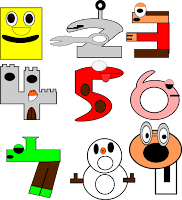Reading and writing numbers might seem intuitive and easy to us as adults, but there is a lot to understand before it becomes second nature to a young child. Think about how you would explain to a child why, in the number 0303, we do not need the first 0 but do need the second one. How would you help a child to really understand that the first 3 has a very different meaning to the second 3? Could you explain why 0.3 and 0.30 are actually the same number? And why can't you always just add a zero when multiplying by 10? The key to a child truly understanding all of these concepts is in allowing them to discover and understand place value and the fact that it is the position of each digit within a number that is important.  (To see a full list of available posts click  here .) In a  previous post , I highlighted how important it is to really understand place value and the way our number system works. The post explains  what place value is, as well as discussing  common mis

Measuring Length - the importance of units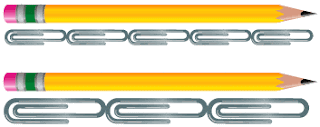In a previous post  I discussed how to introduce the idea of measuring length to young children. The post explored the use of non-standard units (such as Lego bricks), with a particular focus on how and why we need to measure things. In this post I continue with non-standard units, this time demonstrating the importance of unit choice when measuring length. I will evaluate important points to consider when introducing the idea of units, and give some practical, discovery-based task ideas to help provide a deep understanding.

An Early Introduction to MoneyMoney is a fantastic mathematical topic. It is practical, useful to a child's 'real-world' and has links to so many other areas of maths. It can be a difficult topic for a child to fully grasp and will require time and patience, but there are so many fun, engaging and easy to prepare methods to help a child understand money. This post explains how money can link in to other early mathematical topics and provides ideas for tasks that help children really understand money and solve money problems.

Introduction to Measuring Length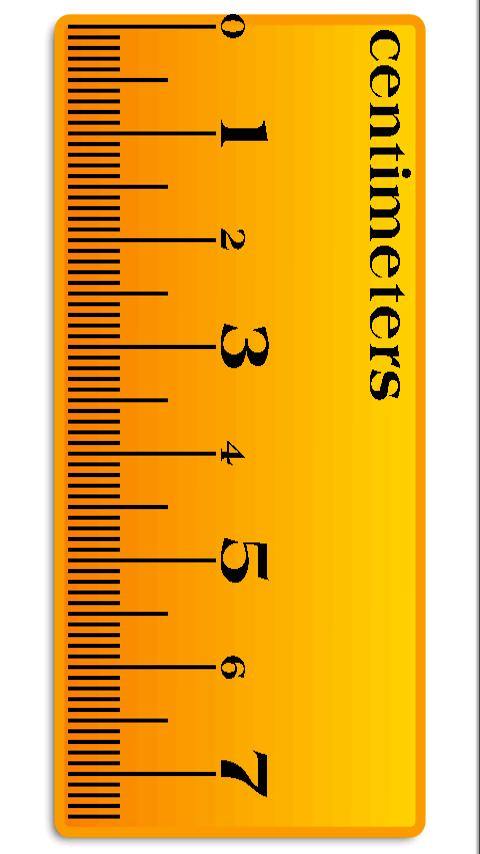I got quite excited about writing this post. Measuring length is a very practical mathematical topic, and so the potential for fun, hands-on maths is endless. I started with the title 'introducing measure' but soon realised that there was too much content for one post. This post, therefore, contains an introduction to measuring length using non-standard units. Posts will soon follow for understanding the importance of units , introducing the standard unit, measuring area, measuring perimeter and an introduction to capacity.

Learning to Tell the Time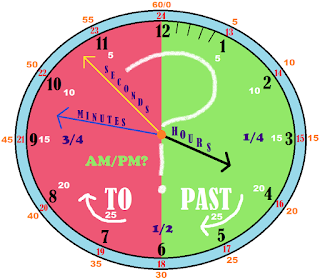Telling the time is a rather abstract concept to begin with and some children find it extremely difficult to get to grips with. To be able to tell the time or solve time problems, children need to have understanding in many different areas of mathematics. Although it can be difficult, if you take it one step at a time, learning to tell the time can be engaging and fun - it is a fantastic mathematical concept that can easily be linked to a child's real-world and time problems can greatly enhance their problem solving skills. This post talks about common misconceptions and difficulties that children may experience. It also highlights different areas of maths that are involved in learning to tell the time and ideas for activities that can be done at home or at school in order to improve a child's time-telling skills.

Really Understand What it Means to Add or SubtractChildren will be adding and subtracting numbers in many contexts throughout their lives. They will need to learn to add and subtract large numbers, decimals, fractions, negatives, algebraic equations and more. However, to be able to carry out these calculations with ease and understanding, students need to really get to grips with and fundamentally understand simple addition and subtraction at a young age. This post covers the early introduction to addition and subtraction with ideas for tasks that help children to really understand the process and the link between the two. To extend children further and develop a deeper understanding, including more on the commutative and associative property of addition (but not subtraction) and the issue with BIDMAS, I will shortly have a new post available.

Early Years Counting - techniques to help kids really understand numbers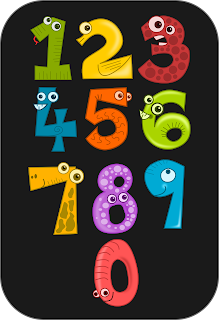I just googled 'learn to count'. The first page is full of online games, videos, activity worksheets and even apps to download. These are all engaging, fun ways for children to practise counting, but they do not teach children what it really means to count. There is much more to counting than is initially obvious. This post explains what is really involved in learning to count and provides ideas of tasks that can be used to really develop a child's understanding of counting.